﻿

### On the number of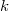$k$-gons in finite projective planes

#### Abstract

Let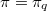$\pi = \pi _q$ denote a finite projective plane of order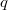$q$,  and let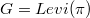$G = Levi (\pi)$ be the bipartite point-line incidence graph of$\pi$.  For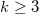$k\ge 3$,  let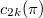$c_{2k} (\pi)$ denote the number of  cycles of length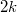$2k$ in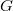$G$.   Are the numbers$c_{2k} (\pi)$ the same for all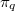$\pi _q$?  We prove that this is the case for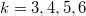$k=3,4,5,6$ by computing these numbers.

DOI Code: 10.1285/i15900932v29n1supplp135

Keywords:
Projective planes; embeddings; k-cycles; Levi graphs

Full Text: PDF